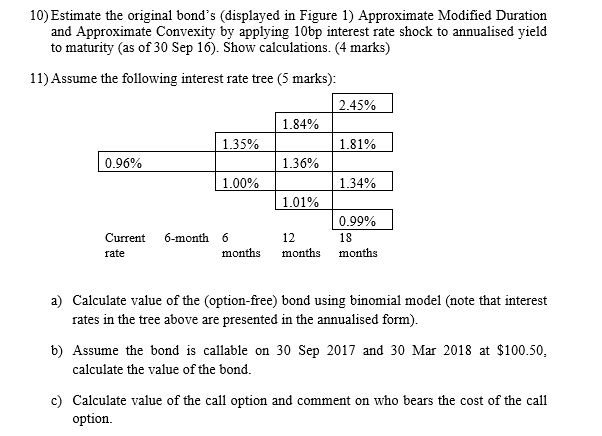# Fixed Income Help me to Solve QuestionsLack of coupon rate and other information about bond.

Hello everyone,

How can we solve this question with a calculator (BA II plus pro)? What are the parameters to enter in my calculator ? Thank you in advance for your help and good luck in your preparation.

“A 5-year, 5% semiannual coupon payment corporate bond is priced at 104.967 per 100 of par value. The bond’s yield-to-maturity, quoted on a semiannual bond basis, is 3.897%. An analyst has been asked to convert to a monthly periodicity. Under this conversion, the yield-to-maturity is closest to:
3.87%.
4.95%.
7.67%.”

First, convert the BEY to a semiannual effective yield:

SAEY = \frac{BEY}{2} = \frac{3.897\%}{2} = 1.9485\%

Next, convert the semiannual effective yield to a monthly effective yield:

\left(1 + MEY\right)^6 = 1 + SAEY = 1.019485
1 + MEY = 1.019485^{1/6} = 1.003221
MEY = 0.003221 = 0.3221\%

Finally, convert the monthly effective yield to an annual (nominal) yield:

Annual\ yield = 0.3221\%\times12 = 3.8657\%

Using the ICONV worksheet on the BAII:

NOM 3.897 C/Y 2 CPT EFF 3.90309257 (the effective rate is now your “anchor”)
C/Y 12 CPT NOM 3.8656328

ETA: You could also use the TVM worksheet

P/Y 2 C/Y 2
N 10 PV -104.967 PMT 2.5 FV 100 CPT I 3.897058409 (matches value in question)
C/Y 12 CPT I 3.8656790324

1 Like

They LET you bring in an approved calculator, it will be your only friend during the exam, ballpoint pens are evil, etc., etc.